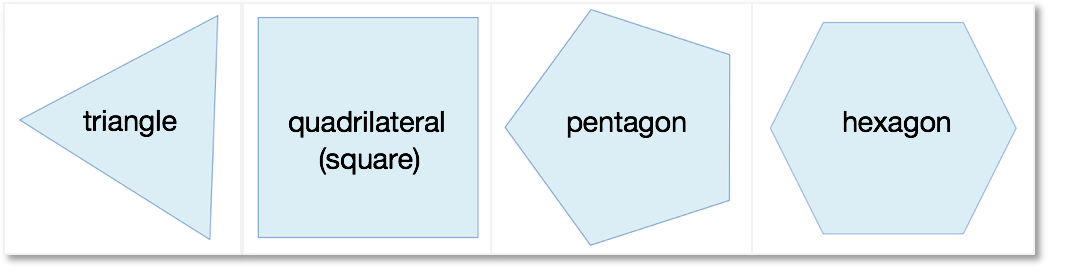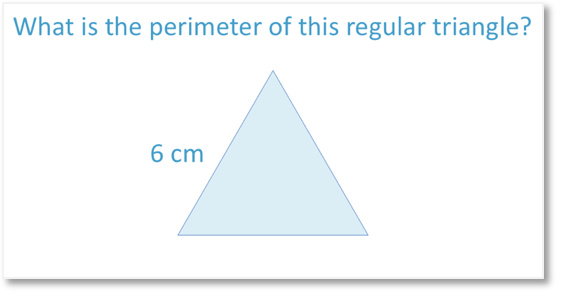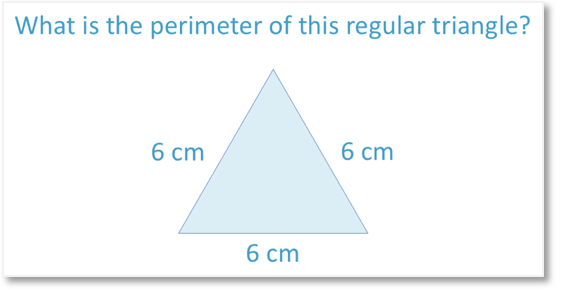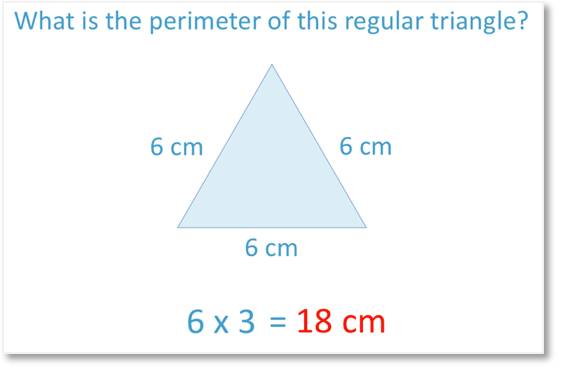# Perimeter of Regular Shapes

Perimeter of Regular Shapes• A regular shape is a shape that has sides that are all the same length as each other and angles that are all the same size as each other.
• The perimeter is the total distance around the outside of a shape.
• To find the perimeter of a regular shape, we simply multiply one side length by how many sides there are.
To find the perimeter of a regular shape we multiply the side length by the number of sides of the shape.

#### List of Regular ShapesAbove is a list of all of the regular shapes from shapes with 3 sides to shapes with 10 sides.

We call a regular triangle an

.

We call a regular

a square.• A square is the special name of a regular quadrilateral.
• A regular quadrilateral is a shape that has four sides of the same length.
• One side length is 12 cm.
• Because a square is regular, all of the other sides are also 12 cm.
• The perimeter is equal to four lots of 12 cm..
• The perimeter of the square is 48 cm.# How to Find the Perimeter of Regular Shapes

To find the perimeter of a regular shape, we multiply the length of one side by the total number of sides.

In this lesson we will use some examples of common regular shapes to explain why.

The definition of a regular shape is a shape in which every side is the same length and every angle is the same size.

Below are some examples of common regular shapes.A regular triangle has the special name of equilateral triangle. We only call triangles that have three equal sides ‘equilateral triangles’.

A regular quadrilateral has the special name of square. We only call quadrilaterals that have four equal sides ‘squares’.

The perimeter of a shape is the total distance around its edge.

Here is an example of finding the perimeter of a regular triangle:

Remember that we might just be told that it is an equilateral triangle.

If a triangle is equilateral it is always regular and its sides are always the same.Remember that ‘regular’ means that all of the sides are the same length.One side is 6 cm long.

Because we are told that our shape is regular, this means that all of the other sides are also 6 cm long.

We have 3 lots of 6 cm.6 x 3 = 18 The perimeter of the equilateral triangle is therefore 18 cm.

The method was to multiply one side length by the total number of sides.

Remember that perimeter is measuring the total length around the outside and because it is a length, our

will be the same as the lengths that we have added up.

We where measuring side lengths in centimetres and so our perimeter units are in centimetres.

Here is another example of finding the perimeter of a regular hexagon:Above, is a regular hexagon.

Because it is a hexagon we know it has six sides.

We just have to remember the names. When teaching regular shape names, it can help us to think that hexagon contains a letter ‘x’ and six is the only number up to ten that also contains a letter ‘x’.

We are told that the hexagon is regular, which means that each of its 6 sides are the same length.

Remember that to find the perimeter of a regular shape, we multiply one side length by the total number of sides.

Each side is 9 cm long. We have 6 lots of 9 cm.9 x 6 = 54

The perimeter of this hexagon is therefore 54 cm.

Next, we will look at a worded problem of finding the perimeter of a regular shape.A hexagon has 6 sides.

It is a regular hexagon, which means that each side is the same length.

Therefore, each side is 6 cm long. We have 6 lots of 6 cm.

The method to find the perimeter of a regular shape is just to multiply one side length by the total number of sides.

6 x 6 = 36

The perimeter of the regular hexagon is 36 cm.

Here’s another worded problem example involving a regular pentagon:A pentagon has 5 sides. It is a regular pentagon, which means that each side is the same length.

The total perimeter of the shape is 45 cm and each of the 5 sides must be the same length.

So, we need to share the total perimeter of 45 cm over the 5 sides.

The mathematical word for sharing is dividing.

We therefore divide 45 by 5.

45 ÷ 5 = 9

Each side of the regular pentagon is 9 cm in length.

When teaching this lesson on perimeter of regular shapes, it can be a disadvantage to not know the names of the common regular shapes first of all.

Print our regular shapes list found in the top of the page summary to have handy when going through our lesson video.Now try our lesson on Perimeter of Compound Shapes where we learn how to find the perimeter of more complicated shapes.error: Content is protected !!